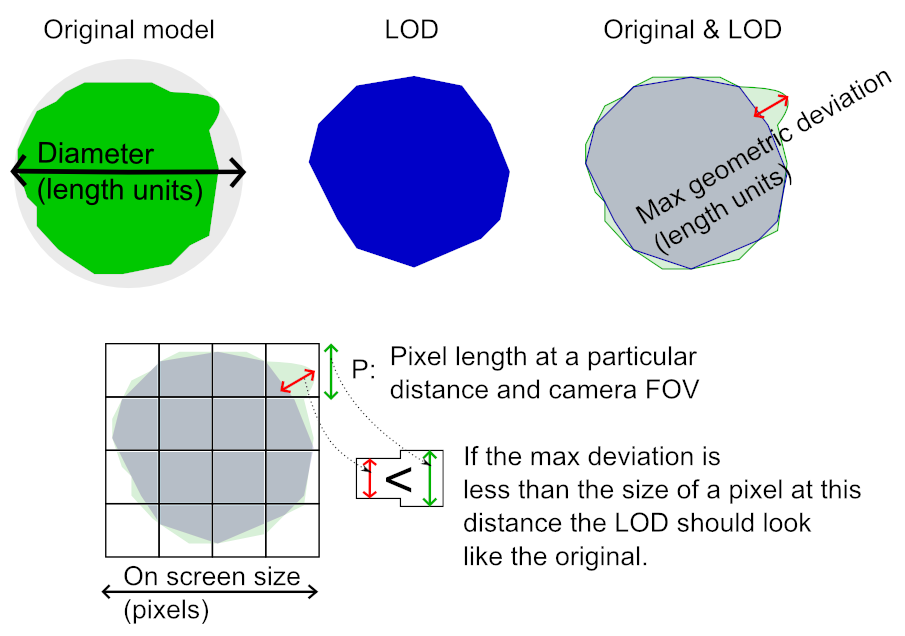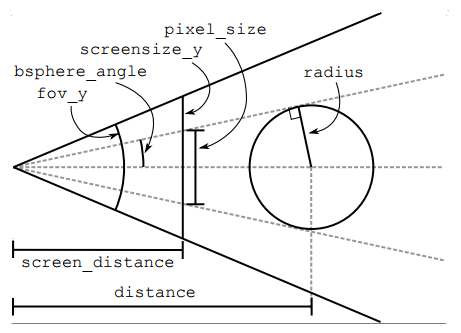# # Deviation and size on screen

Both the reduction and remeshing processor can be set to run until the scene is optimized for a certain amount of pixels on the screen. The remeshing processor has a setting where you simply set the desired on-screen-size, and the reduction processor has a setting where you set the desired maximum allowed deviation (which can be translated to on-screen-size using equation 1).

The amount of pixels is measured along the side of the quadratic screen space bounding box of the scene.

If a LOD is created with on-screen-size set to 100, it should look like the original model at the distance from the camera where it covers at most 100 pixels (in any direction). Any displacement of features (geometric silhouette / texture stretch / normal discrepancies) should be below the visible threshold, i.e. less than the size of a pixel.When a LOD is generated, the deviation of the LOD from the original geometry is measured in number of units (inches, cm or whatever units are used for the scene). This deviation is translated into a recommended on-screen-size (number of pixels). The conversion between on-screen-size and deviation is done as follows:

``````    deviation(length) = scene_bounding_sphere_diameter(length) / on_screen_size(pixels)
``````

For the reduction processor, you can set its maximum allowed deviation. By setting the maximum deviation, you can run a batch of models through the reducer without having to rescale for which on-screen-size you want them fitted to, since that depends on the 3d size of the scene. It is reasonable that you might want to create LODs with similar sized details, if the models are to be viewed at the same distance from the camera.

## # LOD switch distance

To calculate the distance for where the model occupies the particular on screen size, you need the screen resolution, camera FOV and the bounding sphere radius of the model. The calculation goes as follows:

``````// The pixel size of the geometry compared to the number
// of pixels used on the screen.
screen_ratio = pixel_size / screensize_y;
// Normalized distance to the 'screen' if the height of
// the screen is 1.
screen_distance = 1.0 / (tan( Deg2Rad (fov_y/2) );
// The view-angle of the bounding sphere rendered on screen.
bsphere_angle = atan( screen_ratio / screen_distance );
// The distance (along the view vector) to the center of
// the bounding sphere.
distance = radius / sin( bsphere_angle );
``````

In much the same way, the on-screen-size can be calculated using the distance, radius, FOV and screen resolution like this:

``````// The view-angle of the bounding sphere rendered on screen.
bsphere_angle = asin( radius / distance );
// This assumes the near clipping plane is at a
// distance of 1.
geom_view_height = tan( bsphere_angle );
// The size of (half) the screen if the near clipping
// plane is at a distance of 1.
screen_view_height = tan( Deg2Rad (fov_y/2) );
// The ratio of the geometry's screen size compared to
// the actual size of the screen.
view_ratio = geom_view_height / screen_view_height;
// Multiply by the number of pixels on the screen.
pixel_size = view_ratio * screensize_y;
``````

Here is an illustration of distances and angles used for LOD distance calculation (note that the distances have different units).